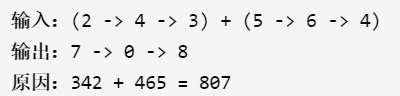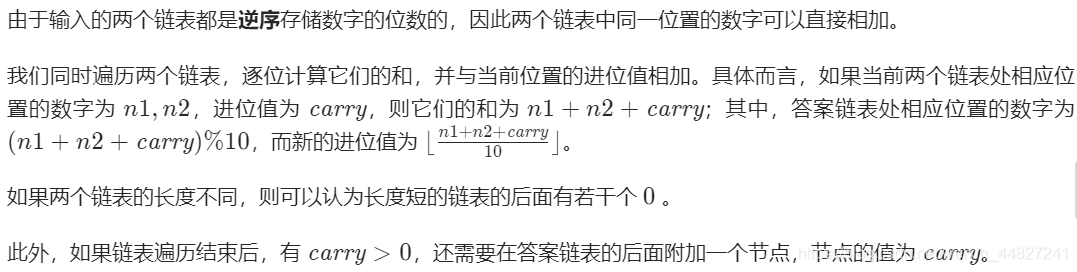# The sum of the two numbers of leetcode

title: gives two non-empty linked lists to represent two non-negative integers. Their individual bits are stored in reverse order, and each of their nodes can only store one digit.
if we add these two Numbers together, a new linked list will be returned to represent their sum.
you can assume that neither number begins with 0, except for the number 0.

example:method: simulate``````class Solution {
public ListNode addTwoNumbers(ListNode l1, ListNode l2) {
ListNode head = null, tail = null;
int carry = 0;
while (l1 != null || l2 != null) {
int n1 = l1 != null ? l1.val : 0;
int n2 = l2 != null ? l2.val : 0;
int sum = n1 + n2 + carry;
if (head == null) {
head = tail = new ListNode(sum % 10);
} else {
tail.next = new ListNode(sum % 10);
tail = tail.next;
}
carry = sum / 10;
if (l1 != null) {
l1 = l1.next;
}
if (l2 != null) {
l2 = l2.next;
}
}
if (carry > 0) {
tail.next = new ListNode(carry);
}